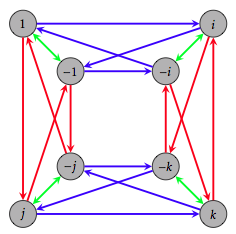The Fall semester of 2013 just ended and one of the classes I taught was abstract algebra. The course is intended to be an introduction to groups and rings, although, I spent a lot more time discussing group theory than the latter. A few weeks into the semester, the students were asked to prove the following theorem.

Theorem. If $G$ is a cyclic group, then all the subgroups of $G$ are cyclic.

As with all conditional theorems, I also encouraged my students to think about whether the converse of this theorem is true. That is, if all the subgroups of a group $G$ are cyclic, does this imply that $G$ is cyclic? Well, since $G$ is always a subgroup of itself, the answer is clearly yes. But this isn’t the interesting question to ask. Instead, we should ask:

If $G$ is a group such that all proper subgroups are cyclic, does this imply that $G$ is cyclic?

The answer is “no” and my students were able to quickly come up with a few counterexamples. In particular, they mentioned the dihedral group $D_3$ (symmetry group for an equilateral triangle), the Klein four-group $V_4$, and the Quarternion group $Q_8$. The groups $D_3$ and $Q_8$ are both non-abelian and hence non-cyclic, but each have 5 subgroups, all of which are cyclic. The group $V_4$ happens to be abelian, but is non-cyclic. Yet it has 4 subgroups, all of which are cyclic.

Following the discussion of these three examples, one of my students asked whether the question above is true for infinite groups. I responded with something like, “Uh…well…no, I don’t think so. Hmmm, let me think about it.” So, I thought about it off and on for a couple hours, but didn’t make much headway. I decided to roam the hallways and recruit some help. I ended up chatting with my colleagues Mike Falk, Jim Swift, and Jeff Rushall. Collectively, we all thought about it a little bit here and a little bit there. I was fairly confident that an internet search would provide some insight, but I was intentionally putting that off in the hopes that I could come up with an example.

A couple days later, I was meeting with one of my undergraduate research students and we chatted briefly about the problem. A few hours after we met, he sent me a link to a discussion on Math Stack Exchange, which contains a response that is precisely about the question above.

Without further ado, here’s an example that confirms that the answer to the question above is “no” even if the group is infinite.

Theorem. The group $G={a/2^k\mid a\in\mathbb{Z}, k\in\mathbb{N}}$ is an infinite non-cyclic group whose proper subgroups are cyclic.

Note that any fixed prime will do for the denominator. Let’s sketch a proof.

First, it is clear that $G$ is an infinite subgroup of $\mathbb{Q}$ since the sum of any two elements from $G$ will be contained in $G$ and the additive inverse of any element from $G$ is also in $G$. To see that $G$ is not cyclic, let $a/2^k\in G$ such that $a$ is odd and consider $\langle a/2^k\rangle$. It’s quickly seen that $\langle a/2^k\rangle$ does not contain any rational numbers having denominators equal to $2^{n}$ for $n>k$, and hence $G$ is not cyclic. Now, suppose that $H$ is a proper subgroup of $G$. If $a/2^k\in H$, then $a/2^{k-1}=a/2^k+a/2^k\in H$, as well. It follows that if there is an element in $H$ with denominator equal $2^k$ (in reduced form), then $H$ also contains elements with denominators equal to $2^n$ (in reduced form) for all $n\leq k$. Since $H$ is a proper subgroup, there exists a smallest $m\in \mathbb{N}$ such that no element of $H$ has a denominator equal to $2^m$. Then it must be the case that $H$ is contained in $\langle 1/2^{m-1}\rangle$, and so $H$ is cyclic (since subgroups of cyclic groups are cyclic).

Cool.

# Dana C. Ernst

Mathematics & Teaching

Northern Arizona University
Flagstaff, AZ
Website
928.523.6852
Instagram
Strava
GitHub
arXiv
ResearchGate
Mendeley
Impact Story
ORCID